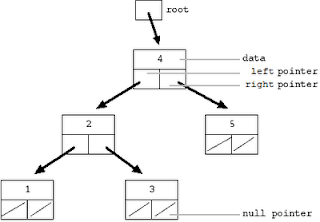Question: Take a binary tree and rearrange left and right pointers to make a circular doubly linked list.

Or

Convert a BST to a sorted circular doubly-linked list in-place.

You are supposed to play with pointers only. Assume left and right pointers of tree as previous and next pointers of doubly-linked list.

Answer: This is one of the most asked problems in programming interviews. Microsoft asks this question very frequently, I was asked the same when I rejected SDE-2 offer from Microsoft a few months ago. You can also find this problem at Stanford CS education library page as The Great Tree List Recursion Problem.

Below is original tree, which we have to convert in a circular doubly linked list.And below is the original tree converted to circular doubly linked list with "next" and "previous" list arrows added.

Above drawing shows the all of the problem state -- the original tree is drawn with plain black lines and the desired next/previous pointers are added in as arrows. Notice that starting with the head pointer.

Looking at the last figure, you might have already started thinking about in-order traversal. If not then please notice that in final linked list (shown below), all the nodes left to a node were actually the nodes from left-sub-tree in original tree. Similarly, all the nodes right to a node were actually the nodes from right-sub-tree in original tree. This is similar to in-order traversal of tree:
1. process left sub tree
2. process current node
3. process right sub tree.
Here extra task is to modify pointers accordingly to create a link list.As we traverse the tree in-order, we can modify a node’s left pointer to point to its predecessor. We also have to modify the predecessor’s right pointer to point to the current node to maintain the doubly linked list behavior.

In this manner, we will be creating a list but that won’t be circular. We can solve this problem by creating a circular doubly linked list at every step in place of creating only doubly linked list. For this, we need to update the current node’s right pointer to point back to the head and the head’s left pointer to point to current node in each recursive call. As the recursion ends, the list’s head and tail would be automatically updated with the correct pointers.

Code:
`struct node {   int data;   struct node* left;   struct node* right;};typedef struct node* Node;//root: Current tree node//prev: this pointer should have the address of in-order predecessor of root//head: this denoted head of final link listvoid treeToDoublyList(Node root, Node & prev, Node & head){   if (!root) return;   treeToDoublyList(root->left, prev, head);   // current node's left points to previous node   root->left = prev;   if (prev)       prev->right = root; // previous node's right points to current node   else       head = root;        // if previous is NULL that current node is head   Node right = root->right; //Saving right node   //Now we need to make list created till now as circular   head->left = root;   root->right = head;   //For right-subtree/parent, current node is in-order predecessor   prev = root;   treeToDoublyList(right, prev, head);}//Wrapper functionNode treeToDoublyList(Node root){   Node prev = NULL;   Node head = NULL;   treeToDoublyList(root, prev, head);   return head;}`

Complexity: As this is nothing but in-order traversal with some extra steps at every node, we are traversing each node just once. Hence time complexity is O(n).

Credits: All the images are copied from Stanford CS library page.